Do you like the article?
Share it with others -

Use new possibilities of MetaTrader 5

# MQL5 Programming Basics: Time

26 April 2013, 11:49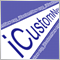2
32 603

### Introduction

MQL5 offers a number of simple functions for working with time and you should not find it difficult getting familiar with them. The range of tasks that require use of date and time is quite small. The main tasks are:

• To perform certain actions at a given point of time (Fig. 1). These may be actions performed at the same time each day or at a given time of the day and a given day of the week on a weekly basis or simply performed at a given date and time.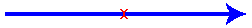Fig. 1. Point of time.

• To enable or disable certain actions within a given time range (time session). This can include a time session within the day (every day from one point of time to another), enabling/disabling certain actions at particular days of the week, time sessions from a given time on one day of the week to a given time on another day of the week and just actions falling within a specified date and time range.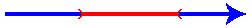Fig. 2. Time range.

In practice, use of time is quite complex. Difficulties are associated with peculiarities of time measurement and the environment where Expert Advisors and indicators operate:

• Missed bars on the chart due to the lack of price changes. They are especially obvious on shorter time frames: M1, M5 and even M15. Missed bars can also be observed on longer time frames.

• Quotes from some dealing centers include Sunday bars that should in fact belong to Monday.

• Weekends. The day of the week preceding Monday is Friday and not Sunday. Friday is followed by Monday, rather than Saturday.

• In addition to Sunday bars, some dealing centers provide quotes continuously, including the entire weekend. Price activity, although quite low compared to weekdays, is present throughout the whole weekend.

• Difference in time zones between the trade server and a local computer (trader's computer and trading terminal). Server time of different dealing centers may vary.

• Daylight Saving Time.

The article will start with some general theory regarding time. Then we will proceed to review the standard MQL5 functions for working with time and concurrently consider some programming techniques, rounding it off with handling practical problems.

The article came out very long, so novice programmers who have only recently begun to explore MQL5 will hardly manage all of it in one go. It would be better to devote it at least three days.

### Peculiarities of Time Measurement

Let's digress from the subject for a little while and turn to astronomy. It is a known fact that the Earth revolves around the Sun, while at the same time rotating on its axis. The Earth's axis is slightly tilted with respect to its orbit around the Sun. The time required for one complete rotation of the Earth on its axis in astronomical (celestial, global) coordinates is called astronomical or sidereal day.

Sidereal day is of no interest to common people on Earth (as opposed to astronomers). More important is the alternation of day and night. The time required for one day-night cycle is called solar day. Looking at the solar system from above the North Pole of the Earth (Fig. 3), you will be able to see that the Earth rotates on its axis and revolves around the Sun counter-clockwise. Therefore, in order to make one complete rotation on its axis with respect to the Sun, the Earth has to rotate a little over 360 degrees. Consequently, a solar day is slightly longer than a sidereal day.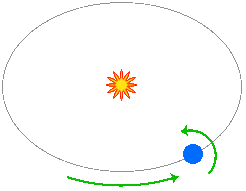Fig. 3. Direction of the Earth's rotation on its axis and around the Sun (as viewed from above the North Pole of the Earth).

For convenience and accuracy, a solar day is taken as the basis of time measurements. A solar day is divided into 24 parts making one hour that lasts 60 minutes, etc. A sidereal day is 23 hours 56 minutes and 4 seconds long. A slight tilt of the Earth's axis relative to its orbital plane results in changes of the seasons noticeable by living beings on the Earth.

The number of solar days in a year is not whole. In fact, it is just a bit over - 365 days and 6 hours. That is why the calendar is adjusted periodically, once every four years (in the year multiple of 4) by adding one more day, the 29th of February (leap year). However this adjustment is not completely accurate (a little extra time is added), so some years, although being multiple of 4, are not leap years. No adjustment of the calendar takes place in the years ending in "00" (a multiple of 100). But that is not all.

If a year is a multiple of 100 and 400 at the same time, such year is considered a leap year and the calendar must be adjusted. Year 1900 is a multiple of 4 and 100, but is not a multiple of 400 which is why it is not a leap year. Year 2000 is a multiple of 4, 100 and 400, making it a leap year. The next year which is a multiple of 4 and 100 is year 2100. But since it is not a multiple of 400, it will not be a leap year. It turns out that the readers of this article leave in the era where every year that is a multiple of 4 is a leap year. The next year that is a multiple of 100 and at the same time a leap year is year 2400.

### Time Zones

The Earth rotates on its axis resulting in the alternation of day and night. It may be day or night, or rather, any time of the day at the same time in different places on the Earth. Since a day has 24 hours, the Earth's circumference is divided into 24 sections of 15 degrees each called time zones. For convenience, the boundaries of time zones do not always follow the longitude lines but instead run in accordance with boundaries of administrative territorial division: borders of countries, regions, etc.

The reference point is the prime meridian called the Greenwich Meridian. It is passing through the London district of Greenwich from which it got its name. Greenwich Mean Time extends 7.5 degrees on either side of the Greenwich meridian. There are 12 time zones measured east from the Greenwich Mean Time (from +1 to +12) and 12 time zones measured west from the Greenwich Mean Time (from -1 to -12). In fact, time zones -12 and +12 are only 7.5 degrees wide instead of 15. Time zones -12 and +12 are located to the right and left of meridian 180, the so-called International Date Line.

Assume, when it is noon at Greenwich (12:00), it is 00:00, i.e. beginning of the day at time zone -12 and - 24:00, i.e. 00:00 of the next day at time zone +12. The same is true about any other hour - while the time on the clock is identical, the dates in the calendar are different. Time zone -12 is in fact not used and is replaced with +12. The same goes for time zone -11 that is substituted by time zone +13. This probably has to do with peculiarities of economic relations: e.g. the Independent State of Samoa located in time zone +13 has strong economic relations with Japan so the time that is closer to Japan time is seen as more convenient.

In addition, there are unusual time zones like -04:30, +05:45, etc. The curious can find the list of all available time zones in Windows time settings.

### Daylight Saving Time

Many countries of the world shift their clocks forward by one hour as a Daylight Saving Time practice in an effort towards a more efficient exploitation of daylight and energy saving. Around 80 countries of the world observe Daylight Saving Time, while others do not. Some countries that use Daylight Saving Time on a wide scale have parts thereof that opt out of the practice (including USA). Daylight Saving Time is observed in major, economically developed countries and regions: almost all European countries (including Germany, UK, Switzerland), USA (New York, Chicago), Australia (Sydney) and New Zealand (Wellington). Japan does not observe Daylight Saving Time. On March 27, 2011, Russia shifted its clocks one hour forward for the last time and never changed back to standard time in October. Since then Russia has been officially out of the Daylight Saving Time practice.

The procedure of changing to a Daylight Saving Time varies from country to country. In the United States, the shift occurs at 02:00 local time on the second Sunday in March and the clock jumps backward at 02:00 on the first Sunday in November. In Europe, clocks shift to Daylight Saving Time at 02:00 on the last Sunday in March and back to standard time at 03:00 on the last Sunday in October. Instead of being set to local time, the shift occurs all at once in all European countries: when it is 02:00 in London, 03:00 in Berlin, etc., according to time zones. Clocks jump backward to standard time when it is 03:00 in London, 04:00 in Berlin, etc.

Australia and New Zealand are located in the southern hemisphere where summer begins when winter comes to the Northern Hemisphere. Consequently, Australia changes time to Daylight Saving on the first Sunday in October and shifts backward to standard time on the first Sunday of April. It is hard to be more precise when it comes to Daylight Saving Time in Australia as start and end dates do not always agree in different parts of the country. In New Zealand, the shift to Daylight Saving Time occurs at 02:00 on the last Sunday in September and jumps backward at 03:00 on the first Sunday in April.

### Time Standard

As mentioned earlier, a solar day is taken as the basis of time measurements and Greenwich Mean Time is taken as the time standard based on which time is determined in all other time zones. Greenwich Mean Time is often abbreviated as GMT.

However, since it was established that the rotation of the Earth is slightly non-uniform, an atomic clock has been used to measure time and UTC (Coordinated Universal Time) has become the new time standard. Currently UTC serves as the primary time standard for the whole world underlying time measurements in all time zones, with necessary adjustments made for Daylight Saving Time. UTC is not subject to Daylight Saving Time.

Due to the fact that solar-based GMT is not completely in line with UTC which is based on atomic clocks, there is a difference between UTC and GMT that builds up to around 1 second about every 500 days. In this regard, a one-second adjustment is performed from time to time on the 30th of June or 31st of December.

### Date and Time Formats

Date formats vary from country to country. In Russia, for example, it is customary to first write the day followed by month and year. Numbers in dates are separated by dots, e.g. 01.12.2012 - December 1, 2012. In USA, the date format is month/day/year, where the numbers in dates are separated by a slash "/". In addition to dots and slashes "/", some format standards may use a dash "-" to separate numbers in a date. When putting down time, hours, minutes and seconds are separated by a colon ":", e.g. 12:15:30 - 12 hours, 15 minutes, 30 seconds.

There is a simple way of specifying date and time format. For example, "dd.mm.yyyy" means that we first write the day (the day of the month consisting of two digits; if the day is a number from 1 to 9, we add 0 at the beginning), then the month (needs to consist of two digits) and the year consisting of four digits. "d-m-yy" means that the day (it can be one-digit number) goes first, followed by the month (one-digit number is allowed) and year consisting of two digits, e.g. 1/12/12 - December 1, 2012. Day, month and year values are separated by a dash "-".

Time is separated from date using a space. The time format uses "h" for hours, "m" for minutes and "s" for seconds, while at the same time specifying the required number of digits. For example, "hh:mi:ss" means that we first write hours (0 should be added in front of values from 1 to 9), then minutes (2 digits necessary) and seconds (2 digits), where values of hours, minutes and seconds are separated by a colon.

The date and time format that is considered to be the most accurate from a programmer's point of view is "yyyy.mm.dd hh:mi:ss". When sorting strings with dates written using this notation, they are easily arranged in chronological order. Suppose, you store information in text files every day and keep them in the same folder. If you name your files using this format, the files in the folder will be conveniently sorted and arranged in order.

Now that we are done with the theoretical part, let us get down to the implementation.

### Time in MQL5

In MQL5, time is measured in seconds elapsed since the beginning of the so-called Unix epoch that began on January 1, 1970. To store time, we use variables of the datetime type. The minimum value of a datetime-type variable is 0 (corresponding to the date of the epoch start), while the maximum value is 32 535 244 799 (corresponding to 23:59:59 on December 31, 3000).

### Determining Current Server Time

To determine the current time, we use the TimeCurrent() function. It returns the last known server time:

```datetime tm=TimeCurrent();
//--- output result

The same server time is used to specify the time of bars in the chart. The last known server time is the time of the last change in the price of any of the symbols opened in the Market Watch window. If there is only EURUSD in the Market Watch window, the TimeCurrent() function will return the last price change of EURUSD. Since the Market Watch window, as a rule, displays a considerable number of symbols, the function basically returns the current server time. However, since prices do not change at the weekend, the value returned by the function will be very much different from the actual server time.

If you need to find out the server time by a certain symbol (time of the last price change), you can use the SymbolInfoInteger() function with the SYMBOL_TIME identifier:

```datetime tm=(datetime)SymbolInfoInteger(_Symbol,SYMBOL_TIME);
//--- output result

### Determining Current Local Time

Local time (displayed by the user's PC clock) is determined by the TimeLocal() function:

```datetime tm=TimeLocal();
//--- output result

In practice, when programming EAs and indicators, you mostly get to use server time. Local time is handy in alerts and journal entries: it is more convenient for a user to compare a message or entry timestamp with the time displayed by the PC clock to see how long ago such message or entry was registered. However, the MetaTrader 5 terminal automatically adds a timestamp to messages and journal entries that are output using the Alert() and Print() functions. Therefore, the need to use the TimeLocal() function may arise only very rarely.

### Time Output

Please note that the value of the tm variable in the above code is output using the Alert() function. That said, the value is displayed in a format that is easy to read, e.g. "2012.12.05 22:31:57". This is due to the fact that the Alert() function converts the arguments passed to it to the string type (the same happens when using the Print() and Comment() function and when outputting to text and csv files). In generating a text message that contains the value of a datetime-type variable, it is your responsibility to perform the type conversion. Convert to the string type if you need to get the formatted time or to the long type followed by the string type if a numerical value is what you need:

```datetime tm=TimeCurrent();
//--- output result

Since value ranges of variables of the long and ulong type cover the range of values of the datetime-type variable, they can also be used to store time but in this case in order to output the formatted time, you need to convert the long type to the datetime type and then to the string type or to the string type alone if you want to output a numerical value:

```long tm=TimeCurrent();
//--- output result

### Time Formatting

Time formatting was considered in the article called "MQL5 Programming Basics: Strings" in the section devoted to converting various variables to a string. Let us briefly outline the key points here. In addition to converting types, MQL5 offers a function that allows you to specify the date and time format when converting them to a string - the TimeToString() function:

```datetime tm=TimeCurrent();
string str1="Date and time with minutes: "+TimeToString(tm);
string str2="Date only: "+TimeToString(tm,TIME_DATE);
string str3="Time with minutes only: "+TimeToString(tm,TIME_MINUTES);
string str4="Time with seconds only: "+TimeToString(tm,TIME_SECONDS);
string str5="Date and time with seconds: "+TimeToString(tm,TIME_DATE|TIME_SECONDS);
//--- output results

The TimeToString() function can be applied to variables of the datetime type, as well as long and ulong type variables and some other types of integer variables but they should not be used to store time.

When formatting a text message using the StringFormat() function, you need to take care of the type conversion.

• When storing time in a datetime type variable:

```datetime tm=TimeCurrent();
//--- generating a string
string str=StringFormat("Formatted: %s, in seconds: %I64i",(string)tm,tm);
//--- output result
• When storing time in a long type variable:

```long tm=TimeCurrent();
//--- generating a string
string str=StringFormat("Formatted: %s, in seconds: %I64i",(string)(datetime)tm,tm);
//--- output result
• Or using the TimeToString() function:

```datetime tm=TimeCurrent();
//--- generating a string
string str=StringFormat("Date: %s",TimeToString(tm,TIME_DATE));
//--- output result

### Converting Time to Numbers. Adding and Subtracting Time

To convert a formatted date (string) to a number (number of seconds elapsed since the beginning of the epoch), the StringToTime() function is used:

```datetime tm=StringToTime("2012.12.05 22:31:57");
//--- output result

As a result of the above code, the output time in seconds will be "1354746717" corresponding to the date as follows "2012.12.05 22:31:57".

When the time is represented as a number, various operations with it become easy and convenient, e.g. you can find the date and time in the past or in the future. Since time is measured in seconds, you should add a period of time expresses in seconds. Knowing that one minute is 60 seconds and one hour is 60 minutes or 3600 seconds, it will not take much effort to calculate the duration of any period of time.

Subtract or add one hour (3600 seconds) from or to the current time and you will get the time that was an hour ago or will be in an hour:

```datetime tm=TimeCurrent();
datetime ltm=tm-3600;
datetime ftm=tm+3600;
//--- output result
Alert("Current: "+(string)tm+", an hour ago: "+(string)ltm+", in an hour: "+(string)ftm);```

The date passed to the StringToTime() function does not have to be complete. Moreover, you can pass the date without the time or time without the date. If you pass the date without the time, the function will return the value at 00:00:00 of the specified date:

```datetime tm=StringToTime("2012.12.05");
//--- output result

If you pass only the time, the function will return the value corresponding to the specified time of the current date:

```datetime tm=StringToTime("22:31:57");
//--- output result

Time can also be passed without seconds. If you pass the date, the only time component that can be passed is hours. This will hardly ever be required in practice. However if you are curious, feel free to experiment with it on your own.

### Date and Time Components

To determine the values of the individual date and time components (year, month, date, etc.), we use the TimeToStruct() function and the MqlDateTime structure. The structure is passed to the function by reference. After executing the function, the structure will be filled with values of the components of the date passed to it:

```datetime    tm=TimeCurrent();
MqlDateTime stm;
TimeToStruct(tm,stm);
//--- output date components
Alert("Day of the year: "  +(string)stm.day_of_year);```

Note that in addition to date components the structure also contains a couple of additional fields: day of the week (the day_of_week field) and day of the year (day_of_year). Days of the week are counted from 0 (0 - Sunday, 1 - Monday, etc.). Days of the year are also counted from zero. The other values follow the generally accepted counting order (months are counted from 1, as well as days).

There is another way of calling the TimeCurrent() function. A structure of the MqlDateTime type is passed to the function by reference. Following the function execution, the structure will be filled with the current date components:

```MqlDateTime stm;
datetime tm=TimeCurrent(stm);
//--- output date components
Alert("Day of the year: "  +(string)stm.day_of_year);```

The TimeLocal() function can also be called in this manner.

### Generating Date from Its Components

You can also reverse transform the MqlDateTime structure to the datetime type. For this purpose, we use the StructToTime() function.

Let us determine the time that was exactly a month ago. The number of days in a month varies. There are months that have 30 or 31 days and February may be 28 or 29 days long. The method of time subtraction and addition that we considered earlier is therefore not quite appropriate. So we will break the dates down into their components, decrease the month value by 1 and if the month value is 1, we will set it to 12 and decrease the year value by 1:

```datetime tm=TimeCurrent();
MqlDateTime stm;
TimeToStruct(tm,stm);
if(stm.mon==1)
{
stm.mon=12;
stm.year--;
}
else
{
stm.mon--;
}
datetime ltm=StructToTime(stm);
//--- output result
Alert("Current: "+(string)tm+", a month ago: "+(string)ltm);```

### Determining Bar Time

When developing an indicator, MetaEditor automatically creates one of the two versions of the OnCalculate() function.

Version 1:

```int OnCalculate(const int rates_total,
const int prev_calculated,
const datetime &time[],
const double &open[],
const double &high[],
const double &low[],
const double &close[],
const long &tick_volume[],
const long &volume[],
{
return(rates_total);
}```

Version 2:

```int OnCalculate(const int rates_total,
const int prev_calculated,
const int begin,
const double &price[])
{
return(rates_total);
}```

Parameters of the first version of the function include the time[] array whose elements contain the time of all bars.

When using the second version, as well as at all times when programming EAs and arranging access from indicators to the time of bars on other time frames, we use the CopyTime() function. This function exists in three versions. In all the versions, the first two function parameters define the symbol and the chart time frame on which the bar time is determined. The last parameter defines an array that stores the returned values and the two middle parameters depend on the function version used.

CopyTime - Version 1. You need to specify the bar index and the number of elements to be copied:

```//--- variables for function parameters
int start = 0; // bar index
int count = 1; // number of bars
datetime tm[]; // array storing the returned bar time
//--- copy time
CopyTime(_Symbol,PERIOD_D1,start,count,tm);
//--- output result

This example demonstrates the implementation of copying the time of the last bar on the D1 time frame. The function version we use depends on the type of parameters passed to it. The above example features the use of the int type variables, which means that we need to get the time by the bar number for the specified number of bars.

When using the CopyTime() function, the bars are counted from zero from right to left. A dynamic array used for the obtained values (the last parameter) is scaled to the required size by the CopyTime() function itself. You can also use a static array but in this case the array size must strictly correspond to the number of requested elements (the value of the 4th parameter).

It is important to understand the order of the elements in the returned array when the time is obtained from several bars at once. While the bars in the chart are counted from right to left starting with the specified bar, the array elements are arranged from left to right:

```//--- variables for function parameters
int start = 0; // bar index
int count = 2; // number of bars
datetime tm[]; // array storing the returned bar time
//--- copy time
CopyTime(_Symbol,PERIOD_D1,start,count,tm);
//--- output result

As a result of this code, the time of yesterday's bar will be stored in the 0 element of the tm array, while the 1st element will contain the time of today's bar.

In some cases, it may appear more convenient to have time in the array arranged in the same order as the order of counting bars in the chart. And here the ArraySetAsSeries() function can do the trick:

```//--- variables for function parameters
int start = 0; // bar index
int count = 2; // number of bars
datetime tm[]; // array storing the returned bar time
ArraySetAsSeries(tm,true); // specify that the array will be arranged in reverse order
//--- copy time
CopyTime(_Symbol,PERIOD_D1,start,count,tm);
//--- output result

Now, the time of today's bar is in the element with index 0 and the time of yesterday's bar is in the element with index 1.

CopyTime - Version 2. Here, when calling the CopyTime() function, we need to specify the time of the bar from which the copying begins and the number of bars to be copied. This version will be appropriate for determining the time of a higher time frame that contains a bar of a lower time frame:

```//--- get the time of the last bar on M5
int m5_start=0;
int m5_count=1;
datetime m5_tm[];
CopyTime(_Symbol,PERIOD_M5,m5_start,m5_count,m5_tm);
//--- determine the bar time on H1 that contains the bar on M5
int h1_count=1;
datetime h1_tm[];
CopyTime(_Symbol,PERIOD_H1,m5_tm,h1_count,h1_tm);
//--- output result
Alert("The bar on М5 with the time "+(string)m5_tm+" is contained in the bar on H1 with the time "+(string)h1_tm);```

It becomes more complicated if you need to determine the time of the bar on a lower time frame, being the beginning of the bar on a higher time frame. A bar with the same time as the time of the bar on a higher time frame may be missing on a lower time frame. In such a case, we will get the time of the last bar on a lower time frame that is contained in the previous bar of the higher time frame. So we need to determine the number of the bar of a lower time frame and get the time of the next bar.

Below is the implementation of the above in the form of a function ready to be used:

```bool LowerTFFirstBarTime(string aSymbol,
ENUM_TIMEFRAMES aLowerTF,
datetime aUpperTFBarTime,
datetime& aLowerTFFirstBarTime)
{
datetime tm[];
//--- determine the bar time on a lower time frame corresponding to the bar time on a higher time frame
if(CopyTime(aSymbol,aLowerTF,aUpperTFBarTime,1,tm)==-1)
{
return(false);
}
if(tm<aUpperTFBarTime)
{
//--- we got the time of the preceding bar
datetime tm2[];
//--- determine the time of the last bar on a lower time frame
if(CopyTime(aSymbol,aLowerTF,0,1,tm2)==-1)
{
return(false);
}
if(tm<tm2)
{
//--- there is a bar following the bar of a lower time frame  that precedes the occurrence of the bar on a higher time frame
int start=Bars(aSymbol,aLowerTF,tm,tm2)-2;
//--- the Bars() function returns the number of bars from the bar with time tm to
//--- the bar with time tm2; since we need to determine the index of the bar following
//--- the bar with time tm2, subtract 2
if(CopyTime(aSymbol,aLowerTF,start,1,tm)==-1)
{
return(false);
}
}
else
{
//--- there is no bar of a lower time frame contained in the bar on a higher time frame
aLowerTFFirstBarTime=0;
return(true);
}
}
//--- assign the obtained value to the variable
aLowerTFFirstBarTime=tm;
return(true);
}```

Function parameters:

• aSymbol - symbol;
• aLowerTF - lower time frame;
• aUpperTFBarTime - bar time on a higher time frame;
• aLowerTFFirstBarTime - returned value of a lower time frame.

All throughout the code, the function checks whether the call of the CopyTime() function is successful and in case of an error, the function returns false. The bar time is returned by reference through the aLowerTFFirstBarTime parameter.

An example of using the function is shown below:

```//--- time of the bar on the higher time frame H1
datetime utftm=StringToTime("2012.12.10 15:00");
//--- variable for the returned value
datetime val;
//--- function call
if(LowerTFFirstBarTime(_Symbol,PERIOD_M5,utftm,val))
{
//--- output result in case of successful function operation
}
else
{
//--- in case of an error, terminate the operation of the function from which the LowerTFFirstBarTime() function is called
return;
}```

A situation where there is no bar on a lower time frame contained in a bar of a higher time frame can arise if the function receives the time of a non-existent bar of a higher time frame. In this case, the function returns true and the time value of 0 is written to the aLowerTFFirstBarTime variable. If a bar of a higher time frame exists, there will always be at least one corresponding bar in each of the lower time frames.

Finding the time of the last bar on a lower time frame that is contained in a bar of a higher time frame is slightly easier. We calculate the time of the next bar on a higher time frame and use the obtained value to determine the time of the corresponding bar on a lower time frame. If the resulting time is equal to the calculated time of a higher time frame, we need to determine the time of the preceding bar on a lower time frame. If the resulting time is less, then we got the right time.

Below is the implementation of the above in the form of a function ready to be used:

```bool LowerTFLastBarTime(string aSymbol,
ENUM_TIMEFRAMES aUpperTF,
ENUM_TIMEFRAMES aLowerTF,
datetime aUpperTFBarTime,
datetime& aLowerTFFirstBarTime)
{
//--- time of the next bar on a higher time frame
datetime NextBarTime=aUpperTFBarTime+PeriodSeconds(aUpperTF);
datetime tm[];
if(CopyTime(aSymbol,aLowerTF,NextBarTime,1,tm)==-1)
{
return(false);
}
if(tm==NextBarTime)
{
//--- There is a bar on a lower time frame corresponding to the time of the next bar on a higher time frame.
//--- Determine the time of the last bar on a lower time frame
datetime tm2[];
if(CopyTime(aSymbol,aLowerTF,0,1,tm2)==-1)
{
return(false);
}
//--- determine the preceding bar index on a lower time frame
int start=Bars(aSymbol,aLowerTF,tm,tm2);
//--- determine the time of this bar
if(CopyTime(aSymbol,aLowerTF,start,1,tm)==-1)
{
return(false);
}
}
//--- assign the obtain value to the variable
aLowerTFFirstBarTime=tm;
return(true);
}```

Function parameters:

• aSymbol - symbol;
• aUpperTF - higher time frame;
• aLowerTF - lower time frame;
• aUpperTFBarTime - bar time on a higher time frame;
• aLowerTFFirstBarTime - returned value of a lower time frame.

All throughout the code, the function checks whether the call of the CopyTime() function is successful and in case of an error, the function returns false. The bar time is returned by reference through the aLowerTFFirstBarTime parameter.

An example of using the function is shown below:

```//--- time of the bar on the higher time frame H1
datetime utftm=StringToTime("2012.12.10 15:00");
//--- variable for the returned value
datetime val;
//--- function call
if(LowerTFLastBarTime(_Symbol,PERIOD_H1,PERIOD_M5,utftm,val))
{
//--- output result in case of successful function operation
}
else
{
//--- in case of an error, terminate the operation of the function from which the LowerTFFirstBarTime() function is called
return;
}```

Attention! It is assumed that the time passed to the function is the exact time of a higher time frame. If the exact time of the bar is unknown and we only know the time of a certain tick on that bar or a bar on a lower time frame contained in the bar on a higher time frame, time normalization will be required. Time frames used in the MetaTrader 5 terminal break the day down into an integral number of bars. We determine the bar number since the beginning of the epoch and multiply it by the length of the bar in seconds:

```datetime BarTimeNormalize(datetime aTime,ENUM_TIMEFRAMES aTimeFrame)
{
int BarLength=PeriodSeconds(aTimeFrame);
return(BarLength*(aTime/BarLength));
}```

Function parameters:

• aTime - time;
• aTimeFrame - time frame.

An example of using the function is shown below:

```//--- the time to be normalized
datetime tm=StringToTime("2012.12.10 15:25");
//--- function call
datetime tm1=BarTimeNormalize(tm,PERIOD_H1);
//--- output result

However, this is not just the time normalization method but also yet another method for determining the time of a higher time frame by the time of a lower time frame.

CopyTime - Version 3. In this case, when calling the CopyTime() function, we specify the time range with respect to bars from which time needs to be copied. This method allows us to easily get all bars of a lower time frame contained in the bar of a higher time frame:

```//--- time of the bar start on H1
datetime TimeStart=StringToTime("2012.12.10 15:00");
//--- the estimated time of the last bar on
//--- M5
datetime TimeStop=TimeStart+PeriodSeconds(PERIOD_H1)-PeriodSeconds(PERIOD_M5);
//--- copy time
datetime tm[];
CopyTime(_Symbol,PERIOD_M5,TimeStart,TimeStop,tm);
//--- output result
Alert("Bars copied: "+(string)ArraySize(tm)+", first bar: "+(string)tm+", last bar: "+(string)tm[ArraySize(tm)-1]);```

### Determining Day Start Time and Amount of Elapsed Time Since the Day Start

The most obvious way of determining the day start time by a given time is to break the time down into its components, zero out hours, minutes and seconds and add them up again. However, there is an easier way. One day is 86400 seconds. We need to get an integer as a result of the division of time by the number of seconds in one day and multiply it by the number of seconds in one day:

```datetime tm=TimeCurrent();
tm=(tm/86400)*86400;
//--- output result

Attention! This trick is only effective with integer variables. If variables of the double and float type appear in any of your calculations, you need to truncate the fractional part using the MathFloor() function:

`MathFloor(tm/86400)*86400`

Following the multiplication, the values should be normalized using the NormalizeDouble() function. Since the resulting value must be an integer, you can use a rounding function, MathRound():

`MathRound(MathFloor(tm/86400)*86400)`

When using integer variables, the remainder is dropped automatically. In dealing with time, there will hardly be any need in variables of the double or float type and their use will most likely indicate a fundamentally wrong approach.

To determine the number of seconds elapsed since the day start, we only need to take the remainder of division of the time by 86400:

```datetime tm=TimeCurrent();
long seconds=tm%86400;
//--- output result
Alert("Time elapsed since the day start: "+(string)seconds+" sec.");```

Similar methods can be used to convert the obtained time in seconds to hours, minutes and seconds. Implementation as a function:

```int TimeFromDayStart(datetime aTime,int &aH,int &aM,int &aS)
{
//--- Number of seconds elapsed since the day start (aTime%86400),
//--- divided by the number of seconds in an hour is the number of hours
aH=(int)((aTime%86400)/3600);
//--- Number of seconds elapsed since the last hour (aTime%3600),
//--- divided by the number of seconds in a minute is the number of minutes
aM=(int)((aTime%3600)/60);
//--- Number of seconds elapsed since the last minute
aS=(int)(aTime%60);
//--- Number of seconds since the day start
return(int(aTime%86400));
}```

The first passed parameter is time. Other parameters are used for the returned values: aH - hours, aM - minutes, aS - seconds. The function itself returns the total number of seconds since the day start. Let us check the function:

```datetime tm=TimeCurrent();
int t,h,m,s;
t=TimeFromDayStart(tm,h,m,s);
//--- output result
Alert("Time elapsed since the day start ",t," s, which makes ",h," h, ",m," m, ",s," s ");```

You can also calculate the number of the day to be used in indicators for determining the bar of the day start:

`bool NewDay=(time[i]/86400)!=(time[i-1]/86400);`

It is assumed that the bars are indexed from left to right, where time[i] is the time of the current bar and time[i-1] is the time of the previous bar.

### Determining Week Start Time and Amount of Elapsed Time Since the Week Start

Determining week start time is a bit more complex than determining the day start. Although the number of days in a week is constant and we can calculate the duration of a week in seconds (604800 seconds), it is not sufficient to simply calculate the integral number of weeks that elapsed since the beginning of the epoch and multiply it by the duration of the week.

The thing is that in the majority of countries the week starts on Monday, while in some other countries (USA, Canada, Israel and other) it starts on Sunday. But as we remember, the epoch of the time measurement starts on Thursday. If Thursday had been the first day of the week, those simple calculations would have sufficed.

For convenience, we will consider the peculiarities of determining the week start through the example of the first epoch day corresponding to the value of 0. We need to find such a value that, when added to the time, would change the first day of the epoch (1970.01.01 00:00), counted from zero, to the fourth day, i.e. we need to add the duration of four days. If the week starts on Monday, Thursday should be the fourth day, so we need to add the duration of three days. If however the week starts on Sunday, Thursday should be the fifth day, so we need to add the duration of four days.

Let us write a function for the calculation of the number of a week:

```long WeekNum(datetime aTime,bool aStartsOnMonday=false)
{
//--- if the week starts on Sunday, add the duration of 4 days (Wednesday+Tuesday+Monday+Sunday),
//    if it starts on Monday, add 3 days (Wednesday, Tuesday, Monday)
if(aStartsOnMonday)
{
aTime+=259200; // duration of three days (86400*3)
}
else
{
aTime+=345600; // duration of four days (86400*4)
}
return(aTime/604800);
}```

This function can be useful in indicators for determining the first bar of the new week:

`bool NewWeek=WeekNum(time[i])!=WeekNum(time[i-1]);`

It is assumed that the bars are indexed from left to right, where time[i] is the time of the current bar and time[i-1] is the time of the previous bar.

Now, we can calculate the time of the week start. Since in order to calculate the number of the week it was assumed that the epoch begins three (or four) days earlier, we now need to perform a reverse time correction:

```long WeekStartTime(datetime aTime,bool aStartsOnMonday=false)
{
long tmp=aTime;
long Corrector;
if(aStartsOnMonday)
{
Corrector=259200; // duration of three days (86400*3)
}
else
{
Corrector=345600; // duration of four days (86400*4)
}
tmp+=Corrector;
tmp=(tmp/604800)*604800;
tmp-=Corrector;
return(tmp);
}  ```

The function returns a value of the long type because the value for the very first week may be negative (three to four days prior to the beginning of the epoch). The second parameter of the function can determine whether the week starts on Sunday or Monday.

Now that we have the time of the week start, we can calculate the number of seconds that elapsed since the week start:

```long SecondsFromWeekStart(datetime aTime,bool aStartsOnMonday=false)
{
return(aTime-WeekStartTime(aTime,aStartsOnMonday));
}```

Seconds can be converted to days, hours, minutes and seconds. While it was not difficult to calculate hours, minutes and seconds since the day start, in this case it will be easier to use the TimeToStruct() function:

```long sfws=SecondsFromWeekStart(TimeCurrent());
MqlDateTime stm;
TimeToStruct(sfws,stm);
stm.day--;
Alert("Time elapsed since the week start "+(string)stm.day+" d, "+(string)stm.hour+" h, "+(string)stm.min+" m, "+(string)stm.sec+" s");```

Please note that the stm.day value is decreased by 1. Days of the month are counted from 1 as we need to determine the number of whole days. Some of you might find the functions of this section useless from a practical standpoint but your understanding of these functions will be of great value as an experience of working with time.

### Determining Week Number Since a Given Date, Year Start or Month Start

Looking at the fields of the MqlDateTime structure, particularly the day_of_year field, one feels like creating functions that will determine the number of the week since the year start and the month start. It is better to write a general function for determining the number of the week since a given date. The principle of the function operation is similar to the one used for determining the number of the week since the beginning of the epoch:

```long WeekNumFromDate(datetime aTime,datetime aStartTime,bool aStartsOnMonday=false)
{
long Time,StartTime,Corrector;
MqlDateTime stm;
Time=aTime;
StartTime=aStartTime;
//--- determine the beginning of the reference epoch
StartTime=(StartTime/86400)*86400;
//--- determine the time that elapsed since the beginning of the reference epoch
Time-=StartTime;
//--- determine the day of the week of the beginning of the reference epoch
TimeToStruct(StartTime,stm);
//--- if the week starts on Monday, numbers of days of the week are decreased by 1,
//    and the day with number 0  becomes a day with number 6
if(aStartsOnMonday)
{
if(stm.day_of_week==0)
{
stm.day_of_week=6;
}
else
{
stm.day_of_week--;
}
}
//--- calculate the value of the time corrector
Corrector=86400*stm.day_of_week;
//--- time correction
Time+=Corrector;
//--- calculate and return the number of the week
return(Time/604800);
}```

Based on this function, let us write two functions for determining the number of the week since the year start and since the month start. To do this, we first need to determine the time of the year start and the time of the month start. Break the time down into its components, adjust values of some fields and convert the components back to time notation.

• The function for determining the time of the year start:

```datetime YearStartTime(datetime aTime)
{
MqlDateTime stm;
TimeToStruct(aTime,stm);
stm.day=1;
stm.mon=1;
stm.hour=0;
stm.min=0;
stm.sec=0;
return(StructToTime(stm));
}```
• The function for determining the time of the month start:

```datetime MonthStartTime(datetime aTime)
{
MqlDateTime stm;
TimeToStruct(aTime,stm);
stm.day=1;
stm.hour=0;
stm.min=0;
stm.sec=0;
return(StructToTime(stm));
}```

Now, below are the functions for determining the number of the week since the year start and month start.

• Since the year start:

```long WeekNumYear(datetime aTime,bool aStartsOnMonday=false)
{
return(WeekNumFromDate(aTime,YearStartTime(aTime),aStartsOnMonday));
}```
• Since the month start:

```long WeekNumMonth(datetime aTime,bool aStartsOnMonday=false)
{
return(WeekNumFromDate(aTime,MonthStartTime(aTime),aStartsOnMonday));
}```

Finally, we get down to purely practical tasks.

### Creating Experimental Tool Set

As already mentioned, there are dealing centers whose quotes include Sunday bars and that provide quotes continuously throughout the entire weekend. We should make sure that the necessary functions operate properly in all cases. We can of course find the suitable dealing centers in the Internet and test the operation of the functions using quotes on demo accounts. However, apart from searching for a right dealing center, we will have to search for appropriate places in the chart to run the required tests.

Let us create our own testing area for testing functions. Friday, weekends and Monday are of our particular interest. We will create an array containing the time of Friday, Monday and weekend bars, as required. There will be a total of four options:

1. No weekend bars.
2. Some bars at the end of Sunday, say, 4.
3. Continuous weekend quotes.
4. Saturday bars but no Sunday bars.

To keep the array from growing too big, we will use the H1 time frame. The maximum array size will be 96 elements (24 bars a day by 4 days) and the array itself will fit on the chart when drawn using graphical objects. So we will get something like an indicator buffer with time and iterate over the array in a loop in the manner similar to the first execution of the OnCalculate() function when starting an indicator. Thus, we will be able to visualize the function operation.

This tool is implemented in the form of a script attached to this article (the sTestArea.mq5 file). Preparation is performed in the OnStart() function of the script. The Variant variable at the very beginning of the function code allows you to select one of the four options listed above. Below the OnStart() function, you can see the LikeOnCalculate() function similar to the OnCalculate() function of indicators. This function has two parameters: rates_total - number of bars and time[] - array with time of the bars.

Further, we continue working in this function as though we were writing an indicator. You can set a marker from the function by calling the SetMarker() function. Parameters passed to the SetMarker() function are: bar index, buffer index (row where the marker is displayed) and marker color.

Fig. 4 shows the script performance results, with the Variant variable being equal to 2 and two rows of markers being set under each bar (bars are marked with relevant timestamps). The color set to all chart elements is invisible.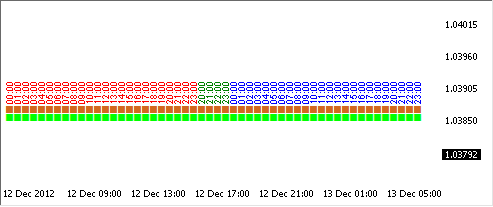Fig. 4. The sTestArea.mq5 script performance.

Bar timestamps take color depending on the day of the week: Friday - red, Saturday - magenta, Sunday - green, Monday - blue. Now, we can proceed to writing various functions that require a special approach to weekend bars, being able to visually monitor their work.

### Pivot Indicator - Option 1

Let us first try to create a simple Pivot indicator. To calculate the Pivot line, we need to know yesterday's closing price, as well as yesterday's maximum and minimum prices. The indicator value is calculated as the mean of these three values. We will identify new highs and lows during the day, calculate the Pivot value at the beginning of the new day and further draw and display the level throughout the day.

We will provide for two versions of the indicator operation:

1. Pivot is calculated every new day (it is assumed that there are no weekend bars). Should there be weekend bars, Saturday and Sunday bars will be treated differently.
2. Saturday bars will belong to Friday, while Sunday bars will belong to Monday (this applies to cases where quotes are provided continuously throughout the weekend and where there are Sunday bars only). Here, you should bear in mind that there may well be no bars at the weekend.

In the first version, it is sufficient to only determine the start of the new day. We pass the current time (aTimeCur) and the previous time (aTimePre) to the function, calculate day numbers since the beginning of the epoch and if they do not match, we deduce that the new day has begun:

```bool NewDay1(datetime aTimeCur,datetime aTimePre)
{
return((aTimeCur/86400)!=(aTimePre/86400));
}```

The second version. If Saturday has started, the day start should be ignored. If Sunday has started, we define the day start (this is naturally just another day). If Monday has started following Sunday, skip the day start. If Monday was preceded by any other day of the week, e.g. Saturday or Friday, define the day start. What we get is a function as follows:

```bool NewDay2(datetime aTimeCur,datetime aTimePre)
{
MqlDateTime stm;
//--- new day
if(NewDay1(aTimeCur,aTimePre))
{
TimeToStruct(aTimeCur,stm);
switch(stm.day_of_week)
{
case 6: // Saturday
return(false);
break;
case 0: // Sunday
return(true);
break;
case 1: // Monday
TimeToStruct(aTimePre,stm);
if(stm.day_of_week!=0)
{ // preceded by any day of the week other than Sunday
return(true);
}
else
{
return(false);
}
break;
default: // any other day of the week
return(true);
}
}
return(false);
}```

And here is the general function depending on the version:

```bool NewDay(datetime aTimeCur,datetime aTimePre,int aVariant=1)
{
switch(aVariant)
{
case 1:
return(NewDay1(aTimeCur,aTimePre));
break;
case 2:
return(NewDay2(aTimeCur,aTimePre));
break;
}
return(false);
}```

Let us test the function operation using the "sTestArea" tool (the attached sTestArea_Pivot1.mq5 file; the day start is marked in brown). You will need to run eight tests: 2 function versions for four bar generation options. Having made sure that the functions operate properly, we can safely start developing an indicator. However, since the development of indicators is not a focus of this article, we have attached hereto a ready made indicator (the Pivot1.mq5 file), with the most difficult part of its development being considered in detail.

### Determining Time Session

We need to allow the Expert Advisor to trade within the specified time range during the day, every day at the same interval. We specify the hours and minutes of the trading session start, as well as the hours and minutes of the trading session end. Hours and minutes specified separately, rather than string variables with the time specified as "14:00" will allow us to perform optimizations in the Strategy Tester if the function is used in the Expert Advisor.

To determine the time session, do as follows:

1. Calculate the time in seconds since the day start for the start point of time and do the same for the end point of time.
2. Calculate the current time in seconds since the day start.
3. Compare the current time with the start and end time.

It is not impossible for a trading session to start on one day and end on another day, i.e. when a trading session goes past midnight, in which case the end time calculated since the day start turns out to be less than the start time. Therefore we need to do two checks. The function we get is as follows:

```bool TimeSession(int aStartHour,int aStartMinute,int aStopHour,int aStopMinute,datetime aTimeCur)
{
//--- session start time
int StartTime=3600*aStartHour+60*aStartMinute;
//--- session end time
int StopTime=3600*aStopHour+60*aStopMinute;
//--- current time in seconds since the day start
aTimeCur=aTimeCur%86400;
if(StopTime<StartTime)
{
//--- going past midnight
if(aTimeCur>=StartTime || aTimeCur<StopTime)
{
return(true);
}
}
else
{
//--- within one day
if(aTimeCur>=StartTime && aTimeCur<StopTime)
{
return(true);
}
}
return(false);
}```

In case the session goes past midnight, the current time should be greater or equal to the session start time OR less than the session end time. If the session takes place within the day, the current time should be greater or equal to the start time AND less than the end time.

An indicator created to test the function operation can be found attached at the end of the article (the Session.mq5 file). Like any other indicator of the application, it can be used not only for testing but also for other practical purposes.

### Determining a Point of Time During the Day

A simple check for equality to the specified time will not work properly as ticks do not occur at regular intervals and there may be delays of several seconds to several minutes. It is very likely that there will simply be no tick with the specified time in the market. We need to check for the intersection of a given timestamp.

The current time should be equal to or greater than the specified time, while the previous time should be less than the specified time. Since it is required to determine a point of time within the day, we need to convert the current time (as well as the previous time) to seconds since the day start. Similarly, the given parameters of time (hours and minutes) should be converted to seconds. It may well be that the previous time falls on the previous day, i.e. it will be greater than the current time, when converted to seconds since the day start. In this case we proceed in the same way as when determining the time session - we do two checks.

The function we get is as follows:

```bool TimeCross(int aHour,int aMinute,datetime aTimeCur,datetime aTimePre)
{
//--- specified time since the day start
datetime PointTime=aHour*3600+aMinute*60;
//--- current time since the day start
aTimeCur=aTimeCur%86400;
//--- previous time since the day start
aTimePre=aTimePre%86400;
if(aTimeCur<aTimePre)
{
//--- going past midnight
if(aTimeCur>=PointTime || aTimePre<PointTime)
{
return(true);
}
}
else
{
if(aTimeCur>=PointTime && aTimePre<PointTime)
{
return(true);
}
}
return(false);
}```

There is an indicator created based on this function (the TimePoint.mq5 file attached to the article).

### Pivot Indicator - Option 2

Now that we have learned how to determine a point of time, let us sophisticate the Pivot indicator. Instead of the usual 00:00, the day will now start at any given time. We will call it a user-defined day. To determine the start of a user-defined day, we will use the earlier described TimeCross() function. Due to the different bar generation options at weekends, some days will have to be omitted. It is difficult to come up with all the checking rules right now, so we will take it one step at a time. Important is to have something to begin with and have options on how to proceed. We have a test script, sTestArea.mq5, so the right solution can even be found experimentally.

The "No weekend bars" case is the most simple: a new day starts at the intersection of a given timestamp by the time.

In case there are only a few bars at the end of Sunday, the TimeCross() function will define the first Sunday bar as the day start, given any function parameters. It is assumed that there are no quotes at weekends (Sunday bars belong to Monday), so Sunday should be ignored. If a given time falls somewhere in the middle of a series of Sunday bars, it should also be ignored as the new day start has already been registered on Friday.

Continuous weekend quotes: If the start of a user-defined day falls in the middle of a calendar day (Fig. 5),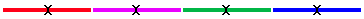Fig. 5. A user-defined day starts in the middle of a calendar day.
Friday - red, Saturday - magenta, Sunday - green, Monday - blue.

a half of Saturday can be treated as Friday and a half of Sunday can be treated as Monday. But there are some bars from the middle of Saturday to the middle of Sunday that do not belong anywhere. We could of course divide the interval from Saturday to Sunday into equal parts and treat one half as Friday and the other half as Monday. This would significantly complicate a very simple indicator, even though weekend quotes are not so important.

The most reasonable solution will be to consider all Saturday and Sunday bars as a user-defined day that lasts from Friday to Monday. This means that the user-defined days that start on Saturday and Sunday are skipped.

The function we get is as follows:

```bool NewCustomDay(int aHour,int aMinute,datetime aTimeCur,datetime aTimePre)
{
MqlDateTime stm;
if(TimeCross(aHour,aMinute,aTimeCur,aTimePre))
{
TimeToStruct(aTimeCur,stm);
if(stm.day_of_week==0 || stm.day_of_week==6)
{
return(false);
}
else
{
return(true);
}
}
return(false);
}```

There is an indicator created based on this function (the Pivot2.mq5 file attached to the article).

### Determining Trading Days of the Week

To allow an Expert Advisor to trade only on certain days is fairly easy. We break the time down into its components using the TimeToStruct() function and declare bool type variables for each of the days of the week in parameters of the Expert Advisor. Depending on the day of the week, the function returns the value of the corresponding variable.

This can be done in a more optimal way. When initializing an Expert Advisor or indicator, fill an array with values of variables that allow or do not allow to trade on certain days. Then check the value of the array element corresponding to the day of the week. We get two functions: one is called during the initialization, while the other is called as required.

Variables:

```input bool Sunday   =true; // Sunday
input bool Monday   =true; // Monday
input bool Tuesday  =true; // Tuesday
input bool Wednesday=true; // Wednesday
input bool Thursday =true; // Thursday
input bool Friday   =true; // Friday
input bool Saturday =true; // Saturday

bool WeekDays;```

The initialization function:

```void WeekDays_Init()
{
WeekDays=Sunday;
WeekDays=Monday;
WeekDays=Tuesday;
WeekDays=Wednesday;
WeekDays=Thursday;
WeekDays=Friday;
WeekDays=Saturday;
}```

The main function:

```bool WeekDays_Check(datetime aTime)
{
MqlDateTime stm;
TimeToStruct(aTime,stm);
return(WeekDays[stm.day_of_week]);
}```

An indicator created based on this function can be found attached at the end of the article (the TradeWeekDays.mq5 file).

### Determining Trading Time of the Week

We need to determine trading sessions from a given time of one day of the week to a given time of another day of the week. This function is similar to the TimeSession() function, with the only difference being that the calculations are based on time elapsed since the week start. The function we get is as follows:

```bool WeekSession(int aStartDay,int aStartHour,int aStartMinute,int aStopDay,int aStopHour,int aStopMinute,datetime aTimeCur)
{
//--- session start time since the week start
int StartTime=aStartDay*86400+3600*aStartHour+60*aStartMinute;
//--- session end time since the week start
int StopTime=aStopDay*86400+3600*aStopHour+60*aStopMinute;
//--- current time in seconds since the week start
long TimeCur=SecondsFromWeekStart(aTimeCur,false);
if(StopTime<StartTime)
{
//--- passing the turn of the week
if(TimeCur>=StartTime || TimeCur<StopTime)
{
return(true);
}
}
else
{
//--- within one week
if(TimeCur>=StartTime && TimeCur<StopTime)
{
return(true);
}
}
return(false);
}```

An indicator created based on this function can be found attached at the end of the article (the SessionWeek.mq5 file).

We have considered virtually all of the most common time-related tasks and reviewed the relevant programming techniques and the standard MQL5 functions required to solve them.

There are some more MQL5 functions for working with time: TimeTradeServer(), TimeGMT(), TimeDaylightSavings() and TimeGMTOffset(). The main peculiarity of these functions is that they are used in the clock and time settings of a user's PC.

The TimeTradeServer() function. It was mentioned earlier in the article that the TimeCurrent() function will show the wrong time at weekends (the time of the last price change on Friday). The TimeTradeServer() function calculates the correct server time:

```datetime tm=TimeTradeServer();
//--- output result

The TimeGMT() function. The function calculates GMT time based on clock values and time settings of a user's computer: time zone and Daylight Saving Time:

```datetime tm=TimeGMT();
//--- output result

To be more precise, the function returns UTC time.

The TimeDaylightSavings() function. The function returns the correction value for Daylight Saving Time from the user's computer settings.

```int val=TimeDaylightSavings();
//--- output result

To get the time without the correction value for Daylight Saving Time, you should add the correction value to the local time.

The TimeGMTOffset() function. The function allows you to get the time zone of a user's computer. The value is returned in seconds to be added to the local time to get GMT time.

```int val=TimeGMTOffset();
//--- output result

The time on a user's computer will be TimeGMT()-TimeGMTOffset()-TimeDaylightSavings():

```datetime tm1=TimeLocal();
datetime tm2=TimeGMT()-TimeGMTOffset()-TimeDaylightSavings();
//--- output result

### Some More Useful Functions for Dealing with Time

Function for determining the leap year

```bool LeapYear(datetime aTime)
{
MqlDateTime stm;
TimeToStruct(aTime,stm);
//--- a multiple of 4
if(stm.year%4==0)
{
//--- a multiple of 100
if(stm.year%100==0)
{
//--- a multiple of 400
if(stm.year%400==0)
{
return(true);
}
}
//--- not a multiple of 100
else
{
return(true);
}
}
return(false);
}```

The principle of determining the leap year is described above in the Peculiarities of Time Measurement section.

Function for determining the number of days in a month

```int DaysInMonth(datetime aTime)
{
MqlDateTime stm;
TimeToStruct(aTime,stm);
if(stm.mon==2)
{
//--- February
if(LeapYear(aTime))
{
//--- February in a leap year
return(29);
}
else
{
//--- February in a non-leap year
return(28);
}
}
else
{
//--- other months
return(31-((stm.mon-1)%7)%2);
}
}```

The function checks whether the year is a leap year to return the appropriate value of either 28 or 29 for February and calculates the number of days for other months. The number of days in the first 7 months alternates as follows: 31, 30, 31, 30, etc., as well as the number of days of the remaining 5 months. Therefore the function calculates the remainder of division by 7. Then we do the odd-parity check and the obtained correction is subtracted from 31.

### Peculiarities of Time Function Operation in the Strategy Tester

The Strategy Tester generates its own stream of quotes and values of the TimeCurrent() function correspond to the stream of quotes in the Strategy Tester. TimeTradeServer() function values correspond to values of TimeCurrent(). Similarly, TimeLocal() function values correspond to values of TimeCurrent(). The TimeCurrent() function in the Strategy Tester does not take into account time zones and the Daylight Saving Time correction. The operation of Expert Advisors is based on price changes, so if your Expert Advisor is required to deal with time, use the TimeCurrent() function. This will allow you to safely test your Expert Advisor in the Strategy Tester.

The TimeGMT(), TimeDaylightSavings() and TimeGMTOffset() functions operate exclusively based on the current settings of a user's computer (shifts to Daylight Saving Time and back are not simulated in the Strategy Tester). If, when testing an Expert Advisor, you need to simulate changes of time to Daylight Saving Time and back to standard time (if this is really necessary), you should take care of it by yourself. This will require information on the exact dates and time for shifting the clock, as well as a thorough analysis.

A solution to this problem is far beyond the scope of a single article and is not considered here. If an Expert Advisor works within the European or American session hours, while the dealing center observes Daylight Saving Time, there will be no discrepancy between the server time and the event time, unlike the Asian session (Japan does not observe Daylight Saving Time and Australia shifts its clocks to Daylight Saving Time in November).

### Conclusion

The article has covered all the standard MQL5 functions for working with time. It set forth the programming techniques used when handling time-related tasks. The article has also demonstrated the creation of several indicators and a few useful functions, with detailed description of their operating principles provided.

All the standard functions for working with time can be classified into several categories:

1. TimeCurrent() and TimeLocal() are the main functions that are used to determine the current time.
2. TimeToString(), StringToTime(), TimeToStruct() and StructToTime() are time processing functions.
3. CopyTime() is a function for working with bar time.
4. TimeTradeServer(), TimeGMT(), TimeDaylightSavings() and TimeGMTOffset() are functions that depend on the user's computer settings.

### Attached Files

• sTestArea.mq5 - a script for testing complex time functions.
• sTestArea_Pivot1.mq5 - the sTestArea.mq5 script used for testing time functions of the Pivot1.mq5 indicator.
• Pivot1.mq5 - a Pivot indicator that uses standard days (the NewDay function).
• Session.mq5 - day's trading session indicator (the TimeSession function).
• TimePoint.mq5 - an indicator of a given point of time (the TimeCross function).
• Pivot2.mq5 - a Pivot indicator that uses user-defined days (the NewCustomDay function).
• TradeWeekDays.mq5 - an indicator of trading days of week (the WeekDays_Check function).
• SessionWeek.mq5 - week's trading session indicator (the WeekSession function).
• TimeFunctions.mqh - all time functions provided in this article, in a single file.

Translated from Russian by MetaQuotes Software Corp.
Original article: https://www.mql5.com/ru/articles/599

Attached files |
stestarea.mq5 (4.96 KB)
pivot1.mq5 (4.5 KB)
session.mq5 (3.51 KB)
timepoint.mq5 (3.32 KB)
pivot2.mq5 (4.14 KB)
sessionweek.mq5 (5.08 KB)
timefuncions.mqh (20.52 KB)
Last comments | Go to discussion (2)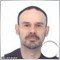| 2 Nov 2015 at 14:41
Thank you so much for this. I learned already the basics of MQL4 and I was searching an article with wich I could begin to learn coding a pivot indicator in MQL5, and your article is very clear. Tank's to you and all publishers of articles.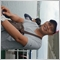| 20 Sep 2019 at 06:57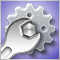Debugging MQL5 Programs

This article is intended primarily for the programmers who have already learned the language but have not fully mastered the program development yet. It reveals some debugging techniques and presents a combined experience of the author and many other programmers.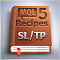MQL5 Cookbook: How to Avoid Errors When Setting/Modifying Trade Levels

In continuation of our work on the Expert Advisor from the previous article of the series called "MQL5 Cookbook: Analyzing Position Properties in the MetaTrader 5 Strategy Tester", we will enhance it with a whole lot of useful functions, as well as improve and optimize the existing ones. The Expert Advisor will this time have external parameters that can be optimized in the MetaTrader 5 Strategy Tester and will in some ways resemble a simple trading system.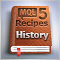MQL5 Cookbook: The History of Deals And Function Library for Getting Position Properties

It is time to briefly summarize the information provided in the previous articles on position properties. In this article, we will create a few additional functions to get the properties that can only be obtained after accessing the history of deals. We will also get familiar with data structures that will allow us to access position and symbol properties in a more convenient way.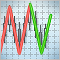The ZigZag Indicator: Fresh Approach and New Solutions

The article examines the possibility of creating an advanced ZigZag indicator. The idea of identifying nodes is based on the use of the Envelopes indicator. We assume that we can find a certain combination of input parameters for a series of Envelopes, whereby all ZigZag nodes lie within the confines of the Envelopes bands. Consequently, we can try to predict the coordinates of the new node.